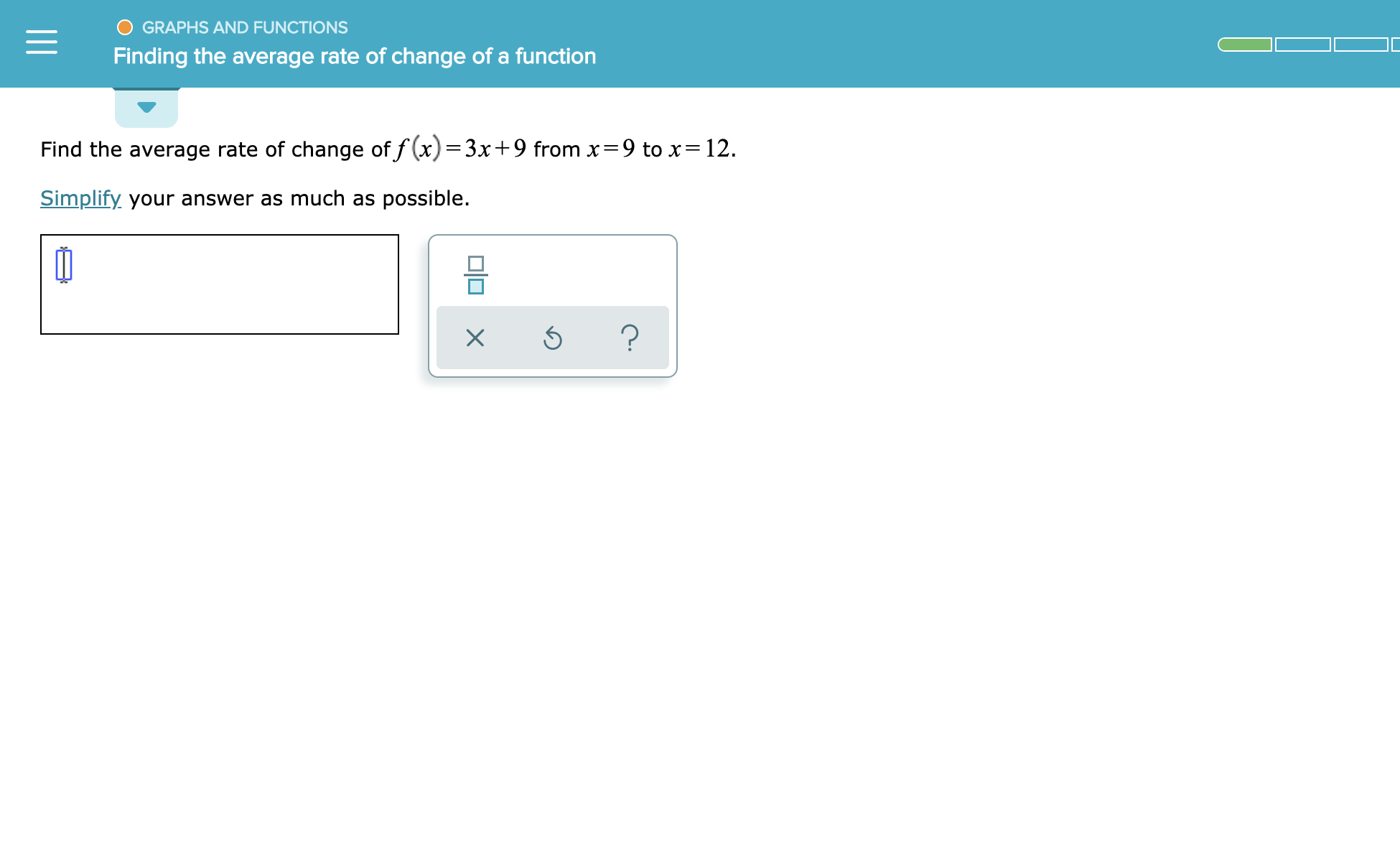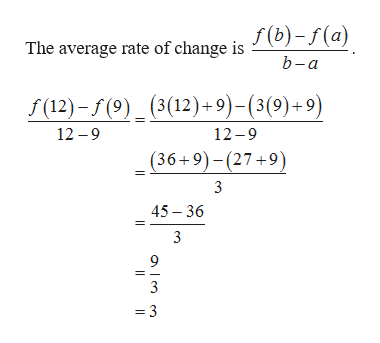GRAPHS AND FUNCTIONSFinding the average rate of change of a functionFind the average rate of change of f(x)=3x+9 from x=9 to x= 12Simplify your answer as much as possible.미미 x

Question

See attachedhelp_outlineImage TranscriptioncloseGRAPHS AND FUNCTIONS Finding the average rate of change of a function Find the average rate of change of f(x)=3x+9 from x=9 to x= 12 Simplify your answer as much as possible. 미미 x fullscreen
Step 1help_outlineImage TranscriptioncloseThe average rate of change is J(b)-f(a) b-a (3(12) 9)-(3(9)+9) f(12)-(9)_ 12-9 12 -9 (36+9)-(27+9) 3 45 36 9 3 =3 fullscreen

Want to see the full answer?

See Solution

Want to see this answer and more?

Our solutions are written by experts, many with advanced degrees, and available 24/7

See Solution
Tagged in

Other